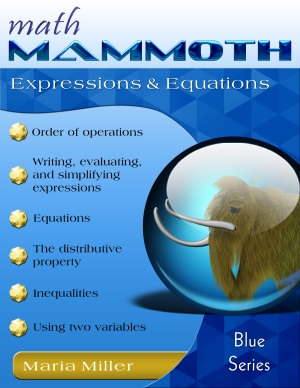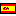^
You are here: HomeBlue Series → Expressions & Equations

# Math Mammoth Expressions & Equations109 pages

## Prices & ordering

Printed copy \$14.25

(credit cards, Google Pay, Paypal, PO)Este libro en español

Math Mammoth Expressions & Equations is a worktext covering the order of operations, equations, expressions, and simplifying expressions in several different ways in 6th-7th grade level.

The main principles are explained and practiced both with visual models and in abstract form, and the lessons contain varying practice problems that approach the concepts from various angles. We also touch on inequalities and graphing on a very introductory level. In order to make the learning of these concepts easier, the expressions and equations in this book do not involve negative numbers (as they typically do when studied in pre-algebra and algebra).

We start out by learning some basic vocabulary used to describe mathematical expressions verbally — terms such as the sum, the difference, the product, the quotient, and the quantity. Next, we study the order of operations. Then, we get into studying expressions in definite terms: students encounter the exact definition of an expression, a variable, and a formula, and practice writing expressions in many different ways.

In the lesson More On Writing and Simplifying Expressions students encounter more terminology: term, coefficient, and constant. In exercise #3, they write an expression for the perimeter of some shapes in two ways. This exercise is once again preparing them to understand the distributive property.

The concepts of equivalent expressions and simplifying expressions are important. If you can simplify an expression in some way, the new expression you get is equivalent to the first. We study these ideas first using lengths — it is a concrete example, and hopefully easy to grasp.

Next, students write and simplify expressions for the area of rectangles and rectangular shapes. After that, the lesson Multiplying and Dividing in Parts leads up to the lessons The Distributive Property 1 and The Distributive Property 2, which concentrate on the symbolic aspect and tying it in with area models.

After studying Properties of the Four Operations, the next topic is equations. Students learn some basics, such as the solutions of an equation are the values of the variables that make the equation true. They use properties of operations and the idea of maintaining the equality of both sides of an equation to solve simple one-step equations. I have also included a few two-step equations as an optional topic.

Lastly, students get to solve and graph simple inequalities, and study the usage of two variables and graphing.

## Better Yet - Bundle Deals!

*BONUS*: Buy any bundle listed above, and get the fully upgraded Soft-Pak software for FREE (includes 4 math and 2 language arts programs, complete with on screen and printable options).

## How and where to order

You can buy Math Mammoth books at:

• Here at MathMammoth.com website — simply use the "Add to cart" buttons you see on the product pages.
• Rainbow Resource carries printed copies for the Light Blue series books, plus several CDs (Light Blue and Blue series).
• At Teachers Pay Teachers you can purchase the Light Blue Series downloads, plus topical units.
• Lulu sells printed copies for most of the Math Mammoth materials (various series).

By purchasing any of the books, permission IS granted for the teacher (or parent) to reproduce this material to be used with his/her students in a teaching situation; not for commercial resale. However, you are not permitted to share the material with another teacher.

In other words, you are permitted to make copies for the students/children you are teaching, but not for other teachers' usage.

Math Mammoth books are PDF files. You will need Adobe Reader to view them, including if you use a Mac or Linux. You can try other PDF viewers, but they seem to either omit or mess up some of the images.
WAIT!

Receive my monthly collection of math tips & resources directly in your inbox — and get a FREE Math Mammoth book!You can unsubscribe at any time.

### Math Mammoth Tour

Confused about the different options? Take a virtual email tour around Math Mammoth! You'll receive:

An initial email to download your GIFT of over 400 free worksheets and sample pages from my books. Six other "TOURSTOP" emails that explain the important things and commonly asked questions concerning Math Mammoth curriculum. (Find out the differences between all these different-colored series!)

This way, you'll have time to digest the information over one or two weeks, plus an opportunity to ask me personally about the curriculum.
A monthly collection of math teaching tips & Math Mammoth updates (unsubscribe any time)### "Mini" Math Teaching Course

This is a little "virtual" 2-week course, where you will receive emails on important topics on teaching math, including:

- How to help a student who is behind
- Troubles with word problems
- Teaching multiplication tables
- Why fractions are so difficult
- The value of mistakes
- Should you use timed tests
- And more!

A GIFT of over 400 free worksheets and sample pages from my books right in the very beginning.A monthly collection of math teaching tips & Math Mammoth updates (unsubscribe any time)
Enter your email to receive math teaching tips, resources, Math Mammoth news & sales, humor, and more! I tend to send out these tips about once monthly, near the beginning of the month, but occasionally you may hear from me twice per month (and sometimes less often).• A GIFT of over 400 free worksheets and sample pages from my books.